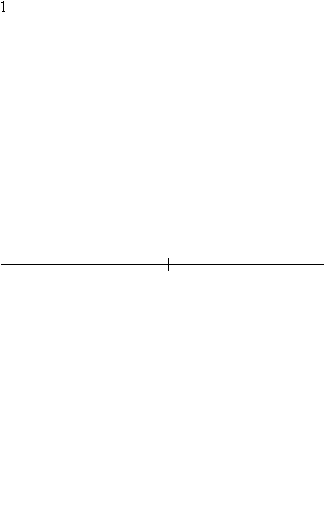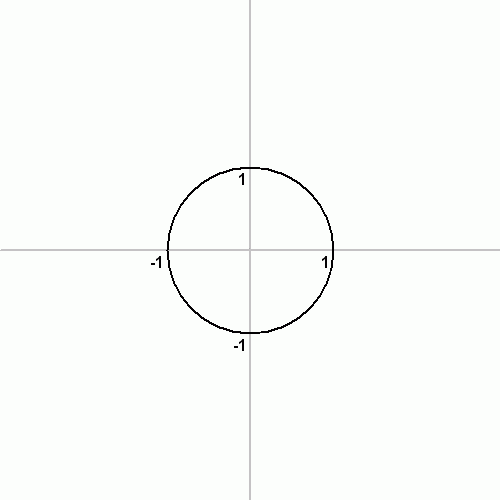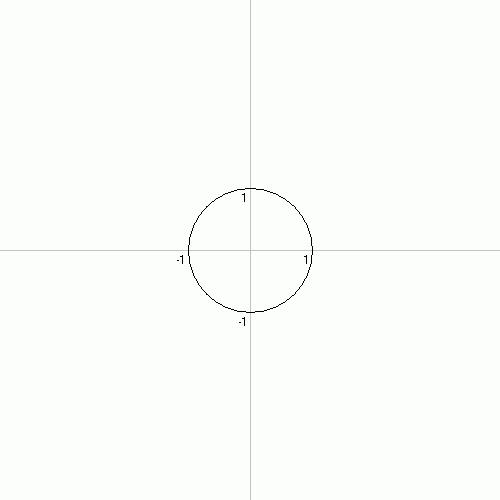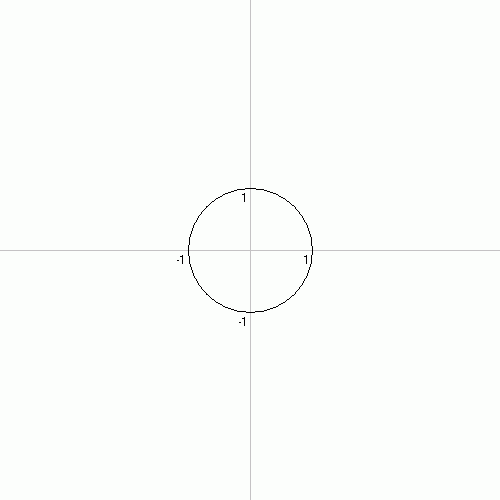# Constructible polygonConstruction of a regular pentagon

In mathematics, a constructible polygon is a regular polygon that can be constructed with compass and straightedge. For example, a regular pentagon is constructible with compass and straightedge while a regular heptagon is not.

## Conditions for constructibilityConstruction of the regular 17-gon

Some regular polygons are easy to construct with compass and straightedge; others are not. The ancient Greek mathematicians knew how to construct a regular polygon with 3, 4, or 5 sides,:p. xi and they knew how to construct a regular polygon with double the number of sides of a given regular polygon.:pp. 49-50 This led to the question being posed: is it possible to construct all regular n-gons with compass and straightedge? If not, which n-gons are constructible and which are not?

Carl Friedrich Gauss proved the constructibility of the regular 17-gon in 1796. Five years later, he developed the theory of Gaussian periods in his Disquisitiones Arithmeticae. This theory allowed him to formulate a sufficient condition for the constructibility of regular polygons:

A regular n-gon can be constructed with compass and straightedge if n is the product of a power of 2 and any number of distinct Fermat primes (including none).

(A Fermat prime is a prime number of the form) Gauss stated without proof that this condition was also necessary, but never published his proof. A full proof of necessity was given by Pierre Wantzel in 1837. The result is known as the Gauss–Wantzel theorem.

Equivalently, a regular n-gon is constructible if and only if the cosine of its common angle is a constructible number—that is, can be written in terms of the four basic arithmetic operations and the extraction of square roots.

### Detailed results by Gauss's theory

Restating the Gauss-Wantzel theorem:

A regular n-gon is constructible with straightedge and compass if and only if n = 2kp1p2...pt where k and t are non-negative integers, and the pi's (when t > 0) are distinct Fermat primes.

The known Fermat primes are:

F0 = 3, F1 = 5, F2 = 17, F3 = 257, and F4 = 65537 (sequence A019434 in the OEIS)

The next twenty-eight Fermat numbers, F5 through F32, are known to be composite.

Thus an n-gon is constructible if

n = 3, 4, 5, 6, 8, 10, 12, 15, 16, 17, 20, 24, 30, 32, 34, 40, 48, 51, 60, 64, 68, 80, 85, 96, 102, 120, 128, 136, 160, 170, 192, 204, 240, 255, 256, 257, 272, 320, 340, 384, 408, 480, 510, 512, 514, 544, 640, 680, 768, 771, 816, 960, 1020, 1024, 1028, 1088, 1280, 1285, 1360, 1536, 1542, 1632, 1920, 2040, 2048, ... (sequence A003401 in the OEIS),

while an n-gon is not constructible with compass and straightedge if

n = 7, 9, 11, 13, 14, 18, 19, 21, 22, 23, 25, 26, 27, 28, 29, 31, 33, 35, 36, 37, 38, 39, 41, 42, 43, 44, 45, 46, 47, 49, 50, 52, 53, 54, 55, 56, 57, 58, 59, 61, 62, 63, 65, 66, 67, 69, 70, 71, 72, 73, 74, 75, 76, 77, 78, 79, 81, 82, 83, 84, 86, 87, 88, 89, 90, 91, 92, 93, 94, 95, 97, 98, 99, 100, 101, 103, 104, 105, 106, 107, 108, 109, 110, 111, 112, 113, 114, 115, 116, 117, 118, 119, 121, 122, 123, 124, 125, 126, 127, ... (sequence A004169 in the OEIS).

### Connection to Pascal's triangle

Since there are 5 known Fermat primes, we know of 31 numbers that are multiples of distinct Fermat primes, and hence 31 constructible odd-sided regular polygons. These are 3, 5, 15, 17, 51, 85, 255, 257, 771, 1285, 3855, 4369, 13107, 21845, 65535, 65537, 196611, 327685, 983055, 1114129, 3342387, 5570645, 16711935, 16843009, 50529027, 84215045, 252645135, 286331153, 858993459, 1431655765, 4294967295 (sequence A045544 in the OEIS). As John Conway commented in The Book of Numbers, these numbers, when written in binary, are equal to the first 32 rows of the modulo-2 Pascal's triangle, minus the top row, which corresponds to monogon. (Because of this, the 1s in such a list form an approximation to the Sierpiński triangle.) This pattern breaks down after this, as the next Fermat number is composite (4294967297 = 641 × 6700417), so the following rows do not correspond to constructible polygons. It is unknown whether any more Fermat primes exist, and is therefore unknown how many odd-sided constructible polygons exist. In general, if there are n Fermat primes, then there are 2n−1 odd-sided constructible polygons.

## General theory

In the light of later work on Galois theory, the principles of these proofs have been clarified. It is straightforward to show from analytic geometry that constructible lengths must come from base lengths by the solution of some sequence of quadratic equations. In terms of field theory, such lengths must be contained in a field extension generated by a tower of quadratic extensions. It follows that a field generated by constructions will always have degree over the base field that is a power of two.

In the specific case of a regular n-gon, the question reduces to the question of constructing a length

cos(2π/n),

which is a trigonometric number and hence an algebraic number. This number lies in the n-th cyclotomic field and in fact in its real subfield, which is a totally real field and a rational vector space of dimension

½φ(n),

where φ(n) is Euler's totient function. Wantzel's result comes down to a calculation showing that φ(n) is a power of 2 precisely in the cases specified.

As for the construction of Gauss, when the Galois group is 2-group it follows that it has a sequence of subgroups of orders

1, 2, 4, 8, ...

that are nested, each in the next (a composition series, in group theory terms), something simple to prove by induction in this case of an abelian group. Therefore there are subfields nested inside the cyclotomic field, each of degree 2 over the one before. Generators for each such field can be written down by Gaussian period theory. For example, for n = 17 there is a period that is a sum of eight roots of unity, one that is a sum of four roots of unity, and one that is the sum of two, which is

cos(2π/17).

Each of those is a root of a quadratic equation in terms of the one before. Moreover these equations have real rather than complex roots, so in principle can be solved by geometric construction: this is because the work all goes on inside a totally real field.

In this way the result of Gauss can be understood in current terms; for actual calculation of the equations to be solved, the periods can be squared and compared with the 'lower' periods, in a quite feasible algorithm.

## Compass and straightedge constructions

Compass and straightedge constructions are known for all known constructible polygons. If n = p·q with p = 2 or p and q coprime, an n-gon can be constructed from a p-gon and a q-gon.

• If p = 2, draw a q-gon and bisect one of its central angles. From this, a 2q-gon can be constructed.
• If p > 2, inscribe a p-gon and a q-gon in the same circle in such a way that they share a vertex. Because p and q are relatively prime, there exists integers a,b such that ap + bq = 1. Then 2aπ/q + 2bπ/p = 2π/pq. From this, a p·q-gon can be constructed.

Thus one only has to find a compass and straightedge construction for n-gons where n is a Fermat prime.

### GalleryFrom left to right, constructions of a 17-gon, 257-gon and 65537-gon. Only the first stage of the 65537-gon construction is shown; the constructions of the 17-gon and 257-gon are given complete.

## Other constructions

The concept of constructibility as discussed in this article applies specifically to compass and straightedge construction. More constructions become possible if other tools are allowed. The so-called neusis constructions, for example, make use of a marked ruler. The constructions are a mathematical idealization and are assumed to be done exactly.

A regular polygon with n sides can be constructed with ruler, compass, and angle trisector if and only ifwhere r, s, k ≥ 0 and where the pi are distinct Pierpont primes greater than 3 (primes of the form:Thm. 2

## References

1. Bold, Benjamin. Famous Problems of Geometry and How to Solve Them, Dover Publications, 1982 (orig. 1969).
2. Fermat factoring status by Wilfrid Keller.
3. Cox, David A. (2012), "Theorem 10.1.6", Galois Theory, Pure and Applied Mathematics (2nd ed.), John Wiley & Sons, p. 259, doi:10.1002/9781118218457, ISBN 978-1-118-07205-9.
4. Magnus Georg Paucker (1822). "Geometrische Verzeichnung des regelmäßigen Siebzehn-Ecks und Zweyhundersiebenundfünfzig-Ecks in den Kreis". Jahresverhandlungen der Kurländischen Gesellschaft für Literatur und Kunst (in German). 2: 160–219.
5. Friedrich Julius Richelot (1832). "De resolutione algebraica aequationis x257 = 1, sive de divisione circuli per bisectionem anguli septies repetitam in partes 257 inter se aequales commentatio coronata". Journal für die reine und angewandte Mathematik (in Latin). 9: 1–26, 146–161, 209–230, 337–358. doi:10.1515/crll.1832.9.337.
6. Johann Gustav Hermes (1894). "Über die Teilung des Kreises in 65537 gleiche Teile". Nachrichten von der Gesellschaft der Wissenschaften zu Göttingen, Mathematisch-Physikalische Klasse (in German). Göttingen. 3: 170–186.
7. Gleason, Andrew Mattei (March 1988). "Angle trisection, the heptagon, and the triskaidecagon" (PDF). The American Mathematical Monthly. 95 (3): 185–194. doi:10.2307/2323624. Archived from the original (PDF) on 2015-12-19.